International
Tables for
Crystallography
Volume B
Reciprocal space
Edited by U. Shmueli

International Tables for Crystallography (2010). Vol. B, ch. 4.6, pp. 591-593   | 1 | 2 |

## Section 4.6.2.2. 1D incommensurately modulated structures

W. Steurera* and T. Haibacha

aLaboratory of Crystallography, Department of Materials, ETH Hönggerberg, HCI G 511, Wolfgang-Pauli-Strasse 10, CH-8093 Zurich, Switzerland
Correspondence e-mail:  walter.steurer@mat.ethz.ch

#### 4.6.2.2. 1D incommensurately modulated structures

| top | pdf |

A periodic deviation of atomic parameters from a reference structure (basic structure, BS) is called a modulated structure (MS). In the case of mutual incommensurability of the basic structure and the modulation period, the structure is called incommensurately modulated. Otherwise, it is called commensurately modulated. The modulated atomic parameters may be one or several of the following:

 (a) coordinates, (b) occupancy factors, (c) thermal displacement parameters, (d) orientation of the magnetic moment.

An incommensurately modulated structure can be described in a dual way by its basic structureand a modulation function. This allows the structure-factor formula to be calculated and a full symmetry characterization employing representation theory to be performed (de Wolff, 1984). A more general method is the nD description: it relates the dD aperiodic incommensurately modulated structure to a periodic structure in nD space. This simplifies the symmetry analysis and structure-factor calculation, and allows more powerful structure-determination techniques.

The nD embedding method is demonstrated in the following 1D example of a displacively modulated structure. A basic structure, with period a and, is modulated by a function, with the satellite vector, period, and α a rational or irrational number yielding a commensurately or incommensurately modulated structure(Fig. 4.6.2.1).Figure 4.6.2.1 | top | pdf |The combination of a basic structure, with period a, and a sinusoidal modulation function, with amplitude A, period λ and, gives a modulated structure (MS). The MS is aperiodic if a and λ are on incommensurate length scales. The filled circles represent atoms.

If the 1D IMS and its 1D modulation function are properly combined in a 2D parameter space, a 2D lattice-periodic structure results (Fig. 4.6.2.2). The actual atoms are generated by the intersection of the 1D physical (external, parallel) spacewith the continuous hyperatoms. The hyper­atoms have the shape of the modulation function along the perpendicular (internal, complementary) space. They result from a convolution of the physical-space atoms with their modulation functions.Figure 4.6.2.2 | top | pdf |2D embedding of the sinusoidally modulated structure illustrated in Fig. 4.6.2.1. The correspondence between the actual displacement of an atom in the 1D structure and the modulation function defined in one additional dimension is illustrated in part (a). Adding to each atom its modulation function in this orthogonal dimension (perpendicular space) yields a periodic arrangement in 2D space V, part (b). The MS results as a special section of the 2D periodic structure along the parallel space. It is obvious from a comparison of (b) and (c) that the actual MS is independent of the perpendicular-space scale.

A basis(D basis) of the 2D hyperlatticeis given bywhere a is the translation period of the BS and c is an arbitrary constant. The components of the basis vectors are given on a 2D orthogonal coordinate system (V basis). The components of the basis vectorare simply the parallel-space period a of the BS and α times the perpendicular-space component of the basis vector. The vectoris always parallel to the perpendicular space and its length is one period of the modulation function in arbitrary units (this is expressed by the arbitrary factor). An atom at position r of the BS is displaced by an amount given by the modulation function, with. Hence, the perpendicular-space variable t has to adopt the value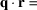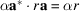for the physical-space variable r. This can be achieved by assigning the slope α to the basis vector. The choice of the parameter c has no influence on the actual MS, i.e. the way in which the 2D structure is cut by the parallel space (Fig. 4.6.2.2c).

The basis of the lattice, reciprocal to Σ, can be obtained from the condition:with. The metric tensors for the reciprocal and direct 2D lattices forareThe choice of an arbitrary number for c has no influence on the metrics of the physical-space components of the IMS in direct or reciprocal space.

The Fourier transform of the hypercrystal depicted in Fig. 4.6.2.2gives the weighted reciprocal lattice shown in Fig. 4.6.2.3. The 1D diffraction patternin physical space is obtained by a projection of the weighted 2D reciprocal latticealongas the Fourier transform of a section in direct space corresponds to a projection in reciprocal space and vice versa: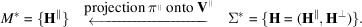Reciprocal-lattice points lying in physical space are referred to as main reflections, all others as satellite reflections. All Bragg reflections can be indexed with integer numbersin the 2D description. In the physical-space description, the diffraction vector can be written as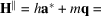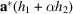, withfor the satellite vector andthe order of the satellite reflection. For a detailed discussion of the embedding and symmetry description of IMSs see, for example, Janssen et al. (2004).Figure 4.6.2.3 | top | pdf |Schematic representation of the 2D reciprocal-space embedding of the 1D sinusoidally modulated structure depicted in Figs. 4.6.2.1and 4.6.2.2. Main reflections are marked by filled circles and satellite reflections by open circles. The sizes of the circles are roughly related to the reflection intensities. The actual 1D diffraction pattern of the 1D MS results from a projection of the 2D reciprocal space onto the parallel space. The correspondence between 2D reciprocal-lattice positions and their projected images is indicated by dashed lines.

A commensurately modulated structure withand,, and with, can be generated by shearing the 2D lattice Σ with a shear matrix:The subscript D (V) following the shear matrix indicates that it is acting on the D (V) basis. The shear matrix does not change the distances between the atoms in the basic structure. In reciprocal space, using the inverted and transposed shear matrix, one obtains### References

Janssen, T., Janner, A., Looijenga-Vos, A. & de Wolff, P. M. (2004). Incommensurate and commensurate modulated crystal structures. In International Tables for Crystallography, Vol. C, edited by E. Prince, ch. 9.8. Dordrecht: Kluwer Academic Publishers.
Wolff, P. M. de (1984). Dualistic interpretation of the symmetry of incommensurate structures. Acta Cryst. A40, 34–42.